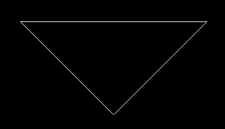Related Articles

# Draw a triangle in C++ graphics

• Last Updated : 02 Sep, 2020

Prerequisite: graphics.h, How to include graphics.h in CodeBlocks?

The task is to write a C program to make a triangle with the line function of graphics.

To run the program we have to include the below header file:

```#include <graphic.h>
```

Approach: The idea is to create a triangle with the help of several lines. We will draw a line in graphics by passing 4 numbers to line() function as:

line(a, b, c, d)
The above function will draw a line from coordinates (a, b) to (c, d) in the output window.

Below is the implementation of the above approach:

## C++

 `// C++ program for drawing a triangle``#include ``#include `` ` `// Driver code``int` `main()``{``    ``// gm is Graphics mode which``    ``// is a computer display``    ``// mode that generates``    ``// image using pixels.``    ``// DETECT is a macro``    ``// defined in "graphics.h"``    ``// header file``    ``int` `gd = DETECT, gm;`` ` `    ``// initgraph initializes``    ``// the graphics system``    ``// by loading a graphics``    ``// driver from disk``    ``initgraph(&gd, &gm, ``""``);`` ` `    ``// Triangle`` ` `    ``// line for x1, y1, x2, y2``    ``line(150, 150, 450, 150);`` ` `    ``// line for x1, y1, x2, y2``    ``line(150, 150, 300, 300);`` ` `    ``// line for x1, y1, x2, y2``    ``line(450, 150, 300, 300);`` ` `    ``// closegraph function closes``    ``// the graphics mode and``    ``// deallocates all memory``    ``// allocated by graphics system``    ``getch();`` ` `    ``// Close the initialized gdriver``    ``closegraph();``}`

Output:
Below is the output of the above program:Want to learn from the best curated videos and practice problems, check out the C++ Foundation Course for Basic to Advanced C++ and C++ STL Course for foundation plus STL.  To complete your preparation from learning a language to DS Algo and many more,  please refer Complete Interview Preparation Course.

My Personal Notes arrow_drop_up